## [mlcourse.ai](mlcourse.ai) – Open Machine Learning Course ###
Author: Nikita Simonov (ODS Slack nick: simanjan)

## Prediction of airlines delay¶

### 1. Feature and data explanation¶

The U.S. Department of Transportation's (DOT) Bureau of Transportation Statistics (BTS) tracks the on-time performance of domestic flights operated by large air carriers. Summary information on the number of on-time, delayed, canceled and diverted flights appears in DOT's monthly Air Travel Consumer Report, published about 30 days after the month's end, as well as in summary tables posted on this website. BTS began collecting details on the causes of flight delays in June 2003. Summary statistics and raw data are made available to the public at the time the Air Travel Consumer Report is released.

This version of the dataset was compiled from the Statistical Computing Statistical Graphics 2009 Data Expo and is also available here. We will consider flight data for 1987.

Import all necessary libraries.

In :
import pandas as pd
import numpy as np
import seaborn as sns
import matplotlib.pyplot as plt

import warnings
warnings.filterwarnings('ignore')


Load dataset. Change path if needed.

In :
raw_data = pd.read_csv("../../data/1987.csv.bz2")

In :
raw_data.head()

Out:
Year Month DayofMonth DayOfWeek DepTime CRSDepTime ArrTime CRSArrTime UniqueCarrier FlightNum ... TaxiIn TaxiOut Cancelled CancellationCode Diverted CarrierDelay WeatherDelay NASDelay SecurityDelay LateAircraftDelay
0 1987 10 14 3 741.0 730 912.0 849 PS 1451 ... NaN NaN 0 NaN 0 NaN NaN NaN NaN NaN
1 1987 10 15 4 729.0 730 903.0 849 PS 1451 ... NaN NaN 0 NaN 0 NaN NaN NaN NaN NaN
2 1987 10 17 6 741.0 730 918.0 849 PS 1451 ... NaN NaN 0 NaN 0 NaN NaN NaN NaN NaN
3 1987 10 18 7 729.0 730 847.0 849 PS 1451 ... NaN NaN 0 NaN 0 NaN NaN NaN NaN NaN
4 1987 10 19 1 749.0 730 922.0 849 PS 1451 ... NaN NaN 0 NaN 0 NaN NaN NaN NaN NaN

5 rows × 29 columns

Variable descriptions

  Name  Description
- Year  1987
- Month 1-12
- DayofMonth    1-31
- DayOfWeek 1 (Monday) - 7 (Sunday)
- DepTime   actual departure time (local, hhmm)
- CRSDepTime    scheduled departure time (local, hhmm)
- ArrTime   actual arrival time (local, hhmm)
- CRSArrTime    scheduled arrival time (local, hhmm)
- UniqueCarrier unique carrier code
- FlightNum flight number
- TailNum   plane tail number
- ActualElapsedTime in minutes
- CRSElapsedTime    in minutes
- AirTime   in minutes
- ArrDelay  arrival delay, in minutes
- DepDelay  departure delay, in minutes
- Origin    origin IATA airport code
- Dest  destination IATA airport code
- Distance  in miles
- TaxiIn    taxi in time, in minutes
- TaxiOut   taxi out time in minutes
- Cancelled was the flight cancelled?
- CancellationCode  reason for cancellation (A = carrier, B = weather, C = NAS, D = security)
- Diverted  1 = yes, 0 = no
- CarrierDelay  in minutes
- WeatherDelay  in minutes
- NASDelay  in minutes
- SecurityDelay in minutes
- LateAircraftDelay in minutes

The target feuture is a 'cancelled'.

### 2. Primary data analysis¶

In :
raw_data.info()

<class 'pandas.core.frame.DataFrame'>
RangeIndex: 1311826 entries, 0 to 1311825
Data columns (total 29 columns):
Year                 1311826 non-null int64
Month                1311826 non-null int64
DayofMonth           1311826 non-null int64
DayOfWeek            1311826 non-null int64
DepTime              1292141 non-null float64
CRSDepTime           1311826 non-null int64
ArrTime              1288326 non-null float64
CRSArrTime           1311826 non-null int64
UniqueCarrier        1311826 non-null object
FlightNum            1311826 non-null int64
TailNum              0 non-null float64
ActualElapsedTime    1288326 non-null float64
CRSElapsedTime       1311826 non-null int64
AirTime              0 non-null float64
ArrDelay             1288326 non-null float64
DepDelay             1292141 non-null float64
Origin               1311826 non-null object
Dest                 1311826 non-null object
Distance             1310811 non-null float64
TaxiIn               0 non-null float64
TaxiOut              0 non-null float64
Cancelled            1311826 non-null int64
CancellationCode     0 non-null float64
Diverted             1311826 non-null int64
CarrierDelay         0 non-null float64
WeatherDelay         0 non-null float64
NASDelay             0 non-null float64
SecurityDelay        0 non-null float64
LateAircraftDelay    0 non-null float64
dtypes: float64(16), int64(10), object(3)
memory usage: 290.2+ MB

In :
raw_data.groupby('Cancelled').size()

Out:
Cancelled
0    1292141
1      19685
dtype: int64
In :
pd.crosstab(raw_data['Month'], raw_data['DayOfWeek'])

Out:
DayOfWeek 1 2 3 4 5 6 7
Month
10 59243 59214 59076 73966 73739 67256 56126
11 73057 58441 58763 55614 54637 52767 69524
12 58411 72583 72396 71331 56537 53347 55798
In :
raw_data.groupby(['UniqueCarrier','FlightNum'])['Distance'].sum().sort_values(ascending=False).iloc[:5]

Out:
UniqueCarrier  FlightNum
AA             2            461628.0
10           447759.0
CO             6            444696.0
AA             874          443726.0
UA             107          443206.0
Name: Distance, dtype: float64

Number of all flights by days of week and months:

### 3. Primary visual data analysis¶

Unique carrier plot.

In :
raw_data.groupby('UniqueCarrier').size().plot(kind='bar');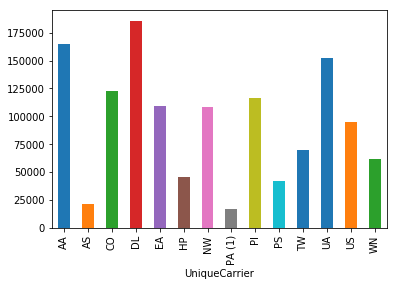Top five of the largest flights by a total distance.

The dataset contains a data for only three month.

Now let's look on the cancelled flights by dayOfweek, dayOfMonth and month.

In :
raw_data[raw_data['Cancelled'] == 1].groupby(['DayOfWeek']).size().plot(kind='bar');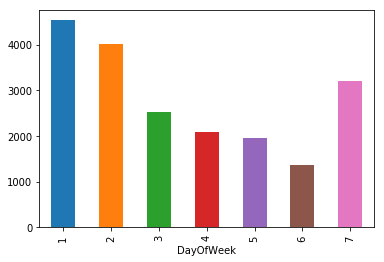In :
raw_data[raw_data['Cancelled'] == 1].groupby(['DayofMonth']).size().plot(kind='bar');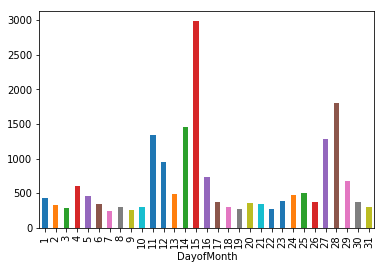In :
raw_data[raw_data['Cancelled'] == 1].groupby(['Month']).size().plot(kind='bar');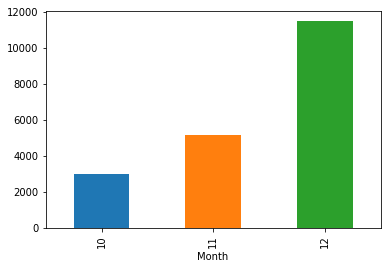Cancelled flights by distance. Here need normalize data for best representation.

In :
fig, ax = plt.subplots(figsize = (12,6))
ax.hist([raw_data['Distance'], raw_data[raw_data['Cancelled'] == 1]['Distance']],
normed=True, label=['All', 'Cancelled'])

ax.set_xlim(0,3000)
ax.set_xlabel('Distance')
ax.set_title('Histogram of Flight Distances')

plt.legend()
plt.show();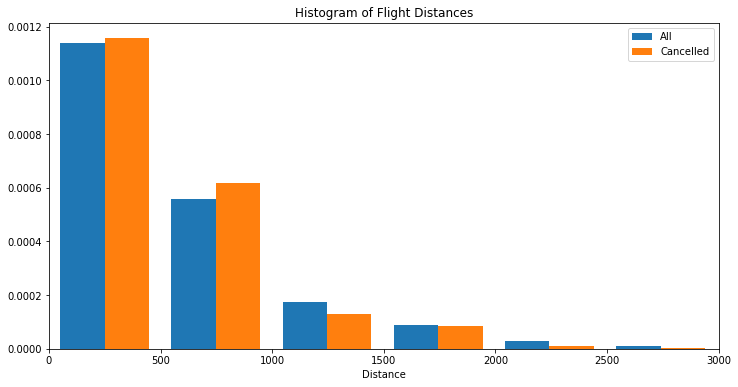Cancelled flights plot UniquerCarrier.

In :
raw_data[raw_data['Cancelled'] == 1].groupby('UniqueCarrier').size().plot(kind='bar');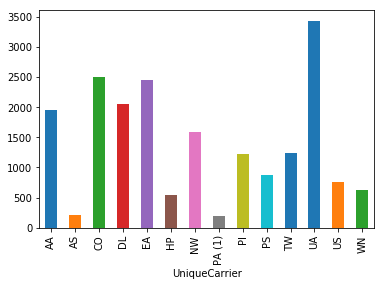Let's look on the top five cancelled flights by Origin and Dest columns.

In :
raw_data[raw_data['Cancelled'] == 1].groupby(['Origin', 'Cancelled']).size()\
.sort_values(ascending=False).iloc[:5].plot(kind='bar');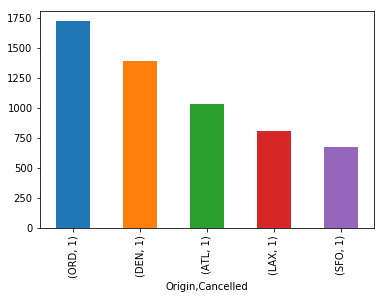In :
raw_data[raw_data['Cancelled'] == 1].groupby(['Dest', 'Cancelled']).size()\
.sort_values(ascending=False).iloc[:5].plot(kind='bar');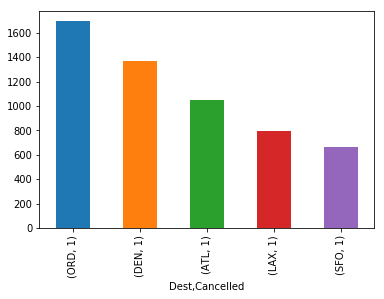In :
fig, ax = plt.subplots(figsize = (12,6))

ax.hist([raw_data['CRSDepTime'], raw_data[raw_data['Cancelled']== 1]['CRSDepTime']], normed=True,
label=['All', 'Cancelled'])

ax.set_xlabel('Scheduled Departure Time')
ax.set_title('Histogram of Scheduled Departure Times')

plt.legend()
plt.show()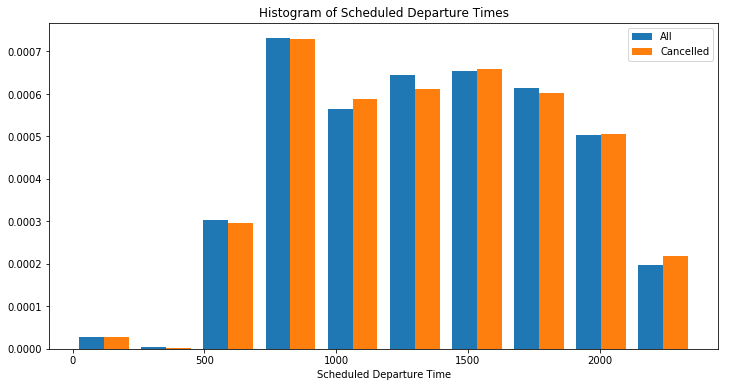Box plot for Distance.

In :
fig, ax = plt.subplots(figsize = (15,6))
sns.boxplot(raw_data['Distance'], ax=ax);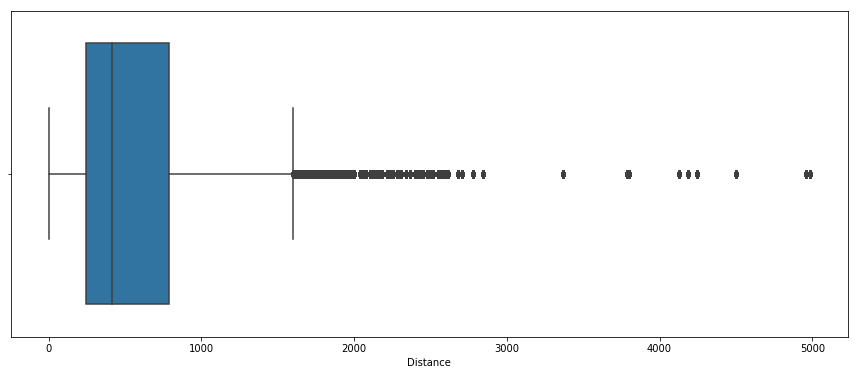The distance column can be scaled for better result.

Building a heatmap for a correlations.

In :
fig, ax = plt.subplots(figsize=(8,8))
sns.heatmap(raw_data.corr(), ax=ax);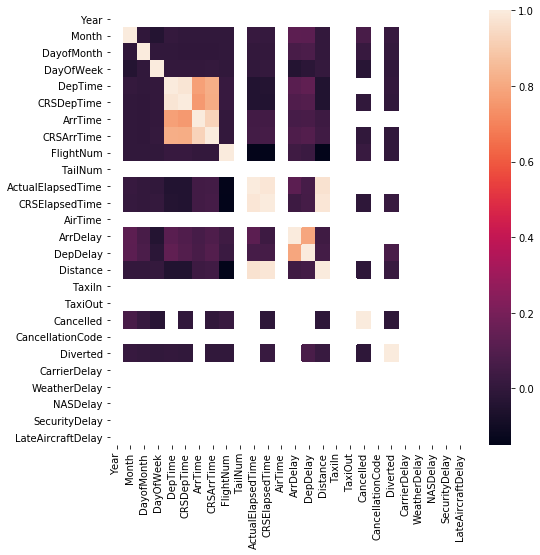### 4. Insights and found dependencies¶

The plots show that there are missing data, as well as some signs correlate with each other.

### 5. Metrics selection¶

Since we have the task of binary 0 or 1 classification as a metric, we can choose:

- Accuracy score.
- Recall score.
- F1 score.
- Precision score.

### 6. Model selection¶

The data contain both binary and categorical features. Therefore, as a model, can choose both a logical regression and a gradient boosting model. We let's see both.

Let's try to building a model.

### 7. Data preprocessing¶

Dropping columns with all NaN values like a 'TailNum' or other.

In :
raw_data.drop(['TailNum', 'AirTime', 'TaxiIn', 'TaxiOut'], axis = 1, inplace=True)


Dropping all delayes columns and also cancellationCode.

In :
raw_data.drop(['CancellationCode', 'CarrierDelay',
'WeatherDelay', 'NASDelay','SecurityDelay', 'LateAircraftDelay'], axis=1, inplace=True)


All columns associated with cancelled flights have a MaN in the some columns, like a 'Carrier Delay', or other delays. Filing it by zero.

In :
raw_data.fillna(0, inplace=True)

In :
raw_data.info()

<class 'pandas.core.frame.DataFrame'>
RangeIndex: 1311826 entries, 0 to 1311825
Data columns (total 19 columns):
Year                 1311826 non-null int64
Month                1311826 non-null int64
DayofMonth           1311826 non-null int64
DayOfWeek            1311826 non-null int64
DepTime              1311826 non-null float64
CRSDepTime           1311826 non-null int64
ArrTime              1311826 non-null float64
CRSArrTime           1311826 non-null int64
UniqueCarrier        1311826 non-null object
FlightNum            1311826 non-null int64
ActualElapsedTime    1311826 non-null float64
CRSElapsedTime       1311826 non-null int64
ArrDelay             1311826 non-null float64
DepDelay             1311826 non-null float64
Origin               1311826 non-null object
Dest                 1311826 non-null object
Distance             1311826 non-null float64
Cancelled            1311826 non-null int64
Diverted             1311826 non-null int64
dtypes: float64(6), int64(10), object(3)
memory usage: 190.2+ MB


Separate the target variable.

In :
raw_data_target = raw_data['Cancelled']
raw_data.drop('Cancelled', axis=1, inplace=True)


Replace Unique Carriers by index.

In :
unique_carrier_list = raw_data['UniqueCarrier'].value_counts().index.tolist()

In :
raw_data['UniqueCarrier'] = raw_data['UniqueCarrier'].apply(lambda x: int(unique_carrier_list.index(x)))


Do the same with Origin and Dest.

In :
origin_list = raw_data['Origin'].value_counts().index.tolist()

In :
raw_data['Origin'] = raw_data['Origin'].apply(lambda x : int(origin_list.index(x)))

In :
dest_list = raw_data['Dest'].value_counts().index.tolist()

In :
raw_data['Dest'] = raw_data['Dest'].apply(lambda x : int(dest_list.index(x)))


Splitting a dataset and training a Logistic Regression on the dataset.

Now let's splitting the data for two parts.

In :
from sklearn.model_selection import train_test_split

In :
X_train, X_test, y_train, y_test = train_test_split(raw_data, raw_data_target, test_size=0.33,
shuffle=True, random_state=17)


### 8. Cross-validation and adjustment of model hyperparameters¶

In :
from catboost import CatBoostClassifier
from sklearn.linear_model import LogisticRegression
from sklearn.model_selection import train_test_split
from sklearn.preprocessing import StandardScaler
from sklearn.metrics import accuracy_score, precision_score, recall_score, f1_score

In :
from sklearn.model_selection import StratifiedKFold

In :
skf = StratifiedKFold(n_splits=5, shuffle=True, random_state=17)

In :
%%time
accuracy_score_list = []
recall_score_list = []
f1_score_list = []
precision_score_list = []

for train_index,test_index in skf.split(raw_data,raw_data_target):
xtr,xvl = raw_data.loc[train_index], raw_data.loc[test_index]
ytr,yvl = raw_data_target.loc[train_index], raw_data_target.loc[test_index]

log_reg = LogisticRegression()
log_reg.fit(xtr,ytr)
predicted = log_reg.predict(xvl)

accuracy_score_list.append(accuracy_score(predicted, yvl))
recall_score_list.append(recall_score(predicted, yvl))
f1_score_list.append(f1_score(predicted, yvl))
precision_score_list.append(accuracy_score(predicted, yvl))

print('Accuracy score mean:{}'.format(np.mean(accuracy_score_list)))
print('Recall score mean:{}'.format(np.mean(recall_score_list)))
print('F1 score mean:{}'.format(np.mean(f1_score_list)))
print('Precision score mean:{}'.format(np.mean(precision_score_list)))

Accuracy score mean:0.9999839917846274
Recall score mean:0.9989345508325471
F1 score mean:0.9994669374089756
Precision score mean:0.9999839917846274
CPU times: user 1min 19s, sys: 2.86 s, total: 1min 22s
Wall time: 1min 21s


Looking at the metrics one can conclude that the target variable in the data has a class imbalance.

Try a CatBoostClassifier.

In :
cat_boost = CatBoostClassifier(random_seed=17, iterations=10)
cat_boost.fit(X_train, y_train, verbose=False, plot=True);


### Part 9. Creation of new features and description of this process¶

Create a new feature 'DepTimeHour' - departure time by hour. Departure time in the night hours can be cause of cancelled flight. Also creating a new features 'Night', 'Morning', 'Afternoon', 'Evening'.

In :
raw_data['DepTimeHour'] = raw_data['CRSDepTime'].apply(lambda x: round(x / 100))

raw_data['Night'] = raw_data['DepTimeHour'].apply(lambda x: int(7 >= x >= 0))
raw_data['Morning'] = raw_data['DepTimeHour'].apply(lambda x:  int(12 >= x > 7))
raw_data['Afternoon'] = raw_data['DepTime'].apply(lambda x: int(18 >= x > 12))
raw_data['Evening'] = raw_data['DepTime'].apply(lambda x: int(23 >= x > 18))


### 10. Plotting training and validation curves¶

In :
from sklearn.metrics import precision_recall_curve, roc_curve, auc

In :
precision, recall, thresholds = precision_recall_curve(y_test, cat_boost.predict(X_test))
thresholds_min = np.argmin(np.abs(thresholds))
closest_zero_p = precision[thresholds_min]
closest_zero_r = recall[thresholds_min]

fig, ax= plt.subplots(figsize=(8,8))
ax.plot(precision, recall, label='Precision-Recall Curve')
ax.plot(closest_zero_p, closest_zero_r)
ax.set_xlabel('Precision')
ax.set_ylabel('Recall')
plt.show()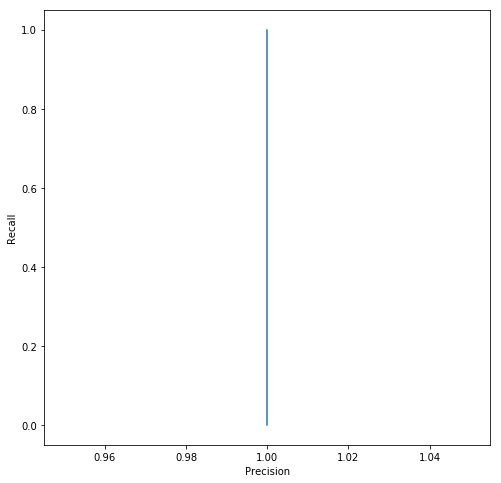### 11. Prediction for test samples¶

In :
print('Accuracy of LogisticRegression :{}'.format(accuracy_score(log_reg.predict(X_test), y_test)))

Accuracy of LogisticRegression :0.9999769001369821

In :
print('Accuracy of Catboost Classifier:{}'.format(accuracy_score(cat_boost.predict(X_test), y_test)))

Accuracy of Catboost Classifier:1.0


### 12. Conclusions¶

The percentage of canceled flights by total.

In :
raw_data_target.value_counts() / raw_data_target.value_counts() * 100

Out:
1.5234405533142281

The models did a great job predicting flight cancellation. After preprocessing the data, the models proved to be even too good. The reason for this was the class imbalance.

The target variable cancelled = 1 is only 1.5 percent by total. In the gradient boosting model, we always predicted the target variable with one hundred percent accuracy, even without fine tuning the model.